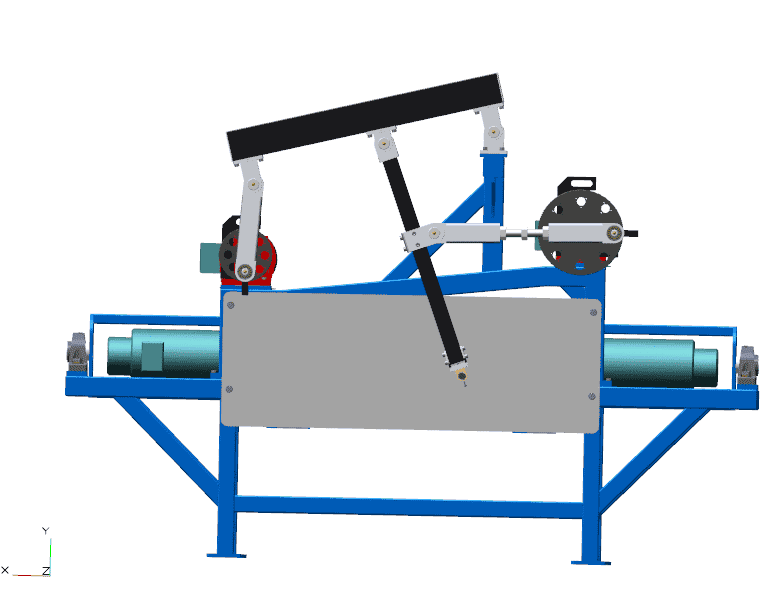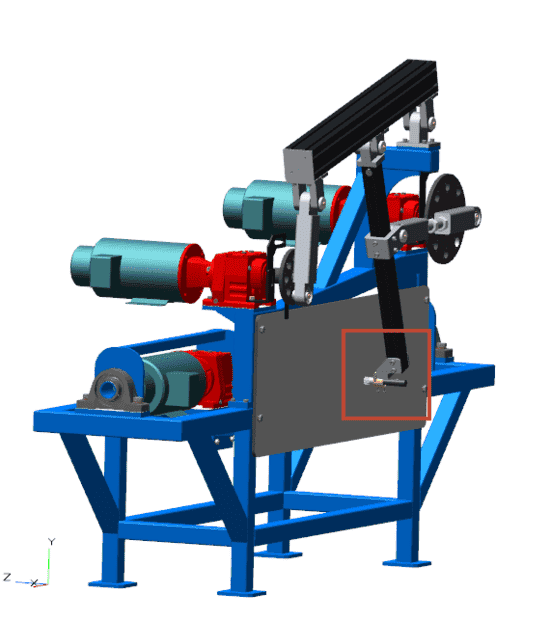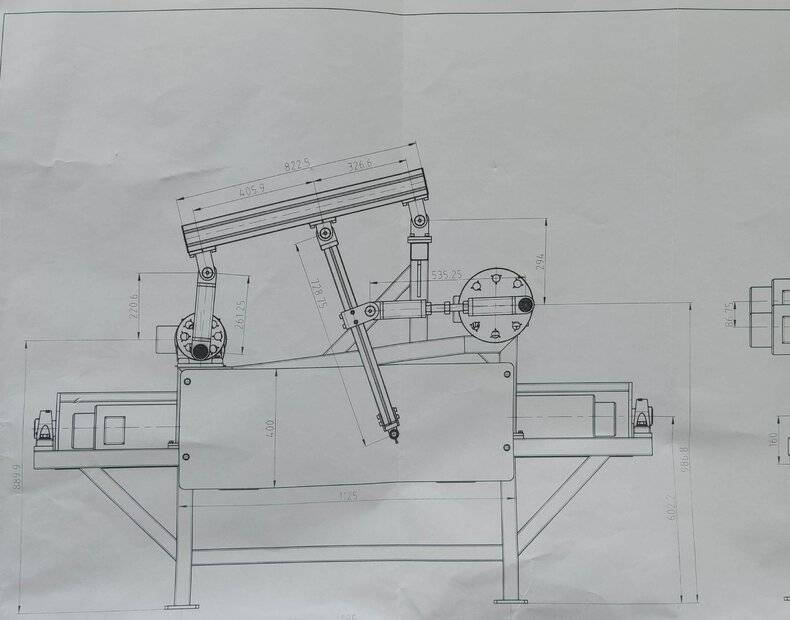# Motor driven pendulum - How to track its position?

• I
• maxitaxi
In summary, the conversation discusses a test pendulum used for rock wool production, driven by two induction motors. The position and speed of the motors at any given time are known, but the movement of the pendulum is currently plotted using a simple analog procedure. The question is how to describe the movement with equations to make it easier to analyze. A suggested solution is to write a program using initial positions and outputs of position and acceleration. It is also recommended to plot the entire linkage at each step for visual confirmation and debugging. Octave is suggested as a free alternative to Matlab for this application.

#### maxitaxi

Hello,

On the image below is a test pendelum for rock wool production. The pendelum is driven by two induction motors. When the right motor makes one revolution, the left motor makes two revolutions. The motors are positionally locked (example: when the right motor is at 35.3° the left motor is at 70.6°). The above part works OK.Currently we are using a simple analog procedure (marker and whiteboard) to plot the position of the pendelum (shown on the image below).On the image below are the mesurements of the moving parts [mm]. The position of both motors at any given time is known. The speed at any given time is also known (nominal speed is 1500 rpm).My question is how can movement of this sort of pendelum (where the marker is located) be described with equations. It would be a lot more transparent and easier to analyze to have the pendelum moevement data in digital form.

Regards.

#### Attachments

I once had a similar problem. It was to design a four bar linkage to follow a specific motion profile while minimizing peak acceleration. I wrote a program in Matlab with initial positions as inputs, and plots of position and acceleration as functions of input shaft position as outputs.

You have more links, but exactly one input (the second motor is geared to the first motor), and one output. It should be an easy program to write:

Calculate position of motor crankpin.
Calculate position of pin between connecting rod and the top beam.
Calculate position of pin in middle of top beam.
Calculate position of crankpin of motor on the right.
Calculate position of pin connecting second motor connecting rod with pendulum.
Calculate position of end of pendulum.
Plot position of pendulum for one revolution of the slower motor.
(Optional) You can use numerical differentiation to calculate velocity and acceleration of the pendulum or any other point in the system.

Hint: It is good practice to plot the entire linkage at each step in the simulation. It slows the simulation down, but you get visual confirmation that the simulation is working properly. It is also helpful for debugging. And it's an excellent tool for showing other people how the mechanism works.

If you do not have Matlab, Octave (https://octave.org/) is Matlab compatible freeware. It's not quite as good as Matlab, but more than good enough for your application.

•hutchphd

## 1. How does a motor driven pendulum work?

A motor driven pendulum works by using a motor to provide a continuous driving force to keep the pendulum oscillating. The motor is connected to the pendulum through a series of gears, which ensures that the driving force is applied at the right time to keep the pendulum moving.

## 2. How is the position of a motor driven pendulum tracked?

The position of a motor driven pendulum can be tracked using a variety of sensors, such as optical sensors or accelerometers. These sensors measure the movement of the pendulum and provide data on its position and velocity.

## 3. What factors affect the accuracy of tracking the position of a motor driven pendulum?

The accuracy of tracking the position of a motor driven pendulum can be affected by various factors, such as the quality of the sensors used, external forces acting on the pendulum, and any friction or resistance in the pendulum's movement.

## 4. How can the position of a motor driven pendulum be improved?

The position of a motor driven pendulum can be improved by using high-quality sensors, minimizing external forces, and reducing friction in the pendulum's movement. Additionally, using a control system to adjust the motor's driving force in real-time based on the position data can also improve accuracy.

## 5. What are the practical applications of a motor driven pendulum?

A motor driven pendulum has various practical applications, such as being used as a precision timekeeping device in clocks and watches, as a sensor in seismometers to measure earthquakes, and as a demonstration tool in physics experiments. It can also be used in engineering and robotics for control and stabilization purposes.